### Home > CALC > Chapter 4 > Lesson 4.2.3 > Problem4-75

4-75.
1.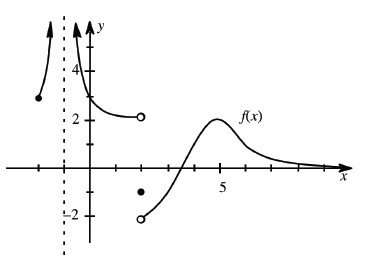The graph of a function f(x) is given at right. Use the graph to evaluate the following limits. Homework Help ✎

1.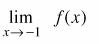2.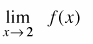3.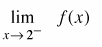4.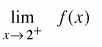5.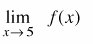6.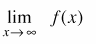7. Where (if anywhere) does the derivative of f(x) not exist?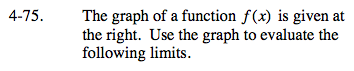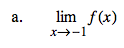The limit does not exist, but y→+∞.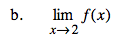A limit is a predicted value (which sometimes differs from the actual function value). The prediction must agree from the left and the right.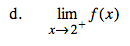What is the prediction from the right?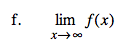lim x→∞ and lim x→−∞ will reveal the equation of a horizontal asymptote, if there is one.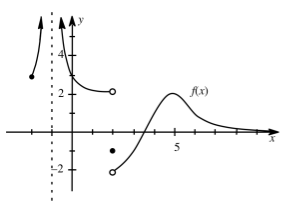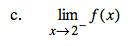What is the prediction from the left?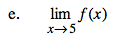Most of the time, the limit and the function value agree.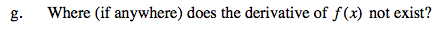Look for cusps, endpoints, jumps, holes, and vertical asymptotes.